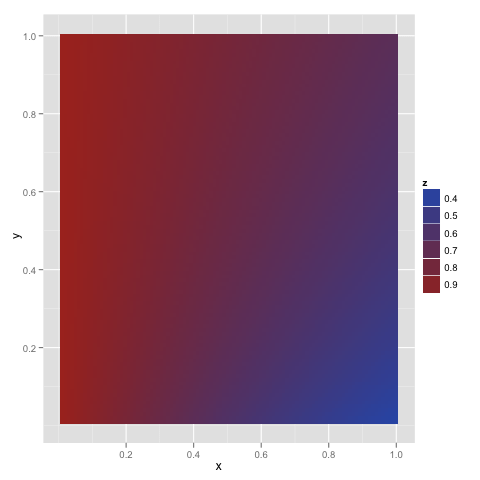Want to share your content on R-bloggers? click here if you have a blog, or here if you don't.

I like exploring the behavior of functions of a single variable using the `curve()` function in R. One thing that seems to be missing from R’s base functions is a tool for exploring functions of two variables.

I asked for examples of such a function on Twitter today and didn’t get any answers, so I decided to build my own. As I see it, there are two ways to visualize a function of two variables:

1. Use a 3D surface.
2. Use a heatmap.

But 3D surfaces aren’t currently available in ggplot2, so I decided to work with heatmaps. The function below provides a very simple implementation of my 3D version of `curve()`, which I call `curve3D()`:

 ```1 2 3 4 5 6 7 8 9 10 ``` ```curve3D <- function(f, from.x, to.x, from.y, to.y, n = 101) { x.seq <- seq(from.x, to.x, (to.x - from.x) / n) y.seq <- seq(from.y, to.y, (to.y - from.y) / n) eval.points <- expand.grid(x.seq, y.seq) names(eval.points) <- c('x', 'y') eval.points <- transform(eval.points, z = apply(eval.points, 1, function (r) {f(r['x'], r['y'])})) p <- ggplot(eval.points, aes(x = x, y = y, fill = z)) + geom_tile() print(p) }```

Here’s an example of the use of `curve3D` to explore the behavior of Loewenstein and Prelec’s Generalized Hyperbolic discounting function:

```g <- function(x, y) {(1 + y * 2) ^ (-x / y) * (1 + y * 1) ^ (x / y)}

curve3D(g, from.x = 0.01, to.x = 1, from.y = 0.01, to.y = 1)
```I'd love suggestions for cleaning this function up. Two obvious improvements are:

1. Allow the function to accept arbitrary expressions and not just functions as inputs.
2. Allow the user to see 3D surfaces or heatmaps.

I suspect that the first problem would be a great way to learn about functional programming in R -- especially R's methods for quoting, parsing and deparsing expressions.